# 索引面试题分析

===============

1 准备数据

1.1 建表

DROP TABLE IF EXISTS test03;
CREATE TABLE test03 (
id INT PRIMARY KEY auto_increment,
c1 char(10),
c2 char(10),
c3 char(10),
c4 char(10),
c5 char(10)
);

1.2 插入数据

INSERT INTO test03(c1, c2, c3, c4, c5) VALUES('a1', 'a2', 'a3', 'a4', 'a5');
INSERT INTO test03(c1, c2, c3, c4, c5) VALUES('b1', 'b2', 'b3', 'b4', 'b5');
INSERT INTO test03(c1, c2, c3, c4, c5) VALUES('c1', 'c2', 'c3', 'c4', 'c5');
INSERT INTO test03(c1, c2, c3, c4, c5) VALUES('d1', 'd2', 'd3', 'd4', 'd5');

2 测试&Explain分析

2.1 建立索引

CREATE INDEX idx_c1234 ON test03(c1, c2, c3, c4);

2.2 测试

Case#1：用到一个索引（注意：key_len=31）

EXPLAIN SELECT * FROM test03 WHERE c1 = 'a1';Case#2：用到两个索引（注意：key_len=62）

EXPLAIN SELECT * FROM test03 WHERE c1 = 'a1' AND c2 = 'a2';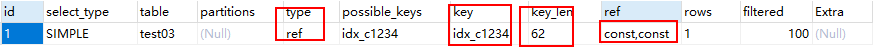Case#3：用到三个索引（注意：key_len=93）

EXPLAIN SELECT * FROM test03 WHERE c1 = 'a1' AND c2 = 'a2' AND c3 = 'a3';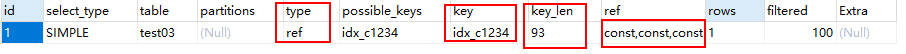Case#4：用到四个索引（注意：key_len=124）

EXPLAIN SELECT * FROM test03 WHERE c1 = 'a1' AND c2 = 'a2' AND c3 = 'a3' AND c4 = 'a4';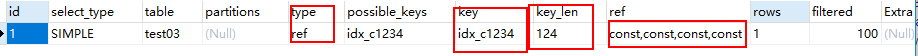Case#5：用到四个索引（注意：这里的查询条件顺序是 1 2 4 3 而不是 1 2 3 4）

EXPLAIN SELECT * FROM test03 WHERE c1 = 'a1' AND c2 = 'a2' AND c4 = 'a4' AND c3 = 'a3';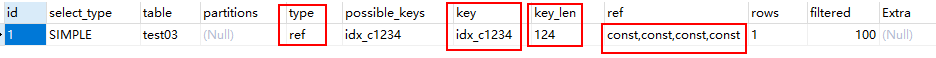Case#6：用到四个索引（这里的顺序是 4 3 2 1）

EXPLAIN SELECT * FROM test03 WHERE c4 = 'a4' AND c3 = 'a3' AND c2 = 'a2' AND c1 = 'a1';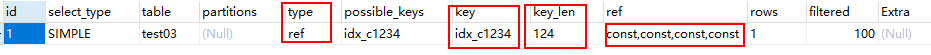Case#7：用到了三个索引（注意：第三个查询条件是大于）

EXPLAIN SELECT * FROM test03 WHERE c1 = 'a1' AND c2 = 'a2' AND c3 > 'a3' AND c4 = 'a4';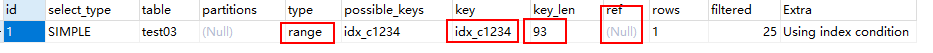• key_len=93
• type=range
• ref=Null
• 由以上三点可推知：使用了三个索引（使用到了 1 2 3 三个索引；由于范围之后全失效，所以第四个索引没有被使用到）

Case#8：用到了四个索引

EXPLAIN SELECT * FROM test03 WHERE c1 = 'a1' AND c2 = 'a2' AND c4 > 'a4' AND c3 = 'a3';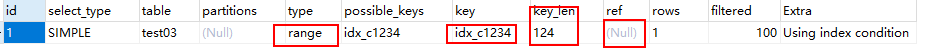• key_len=124
• type=range
• ref=Null
• 使用到了四个索引

Case#9：用到了 1 2 两个索引

EXPLAIN SELECT * FROM test03 WHERE c1 = 'a1' AND c2 = 'a2' ORDER BY c3;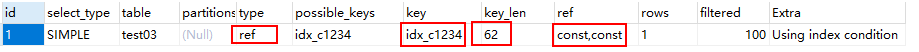Case#10：用到了 1 2 两个索引

EXPLAIN SELECT * FROM test03 WHERE c1 = 'a1' AND c2 = 'a2' AND c4 = 'a4' ORDER BY c3;Case#11：用到了 1 2 两个索引

EXPLAIN SELECT * FROM test03 WHERE c1 = 'a1' AND c2 = 'a2' ORDER BY c4;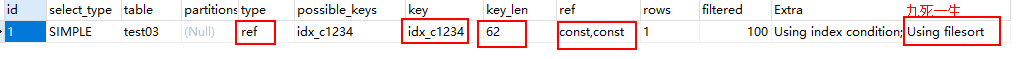Case#12：用到了一个索引

EXPLAIN SELECT * FROM test03 WHERE c1 = 'a1' AND c5 = 'a5' ORDER BY c2, c3;Case#13：用到了一个索引（注意：ORDER BY 后面是 c3 在前，c2 在后）

EXPLAIN SELECT * FROM test03 WHERE c1 = 'a1' AND c5 = 'a5' ORDER BY c3, c2;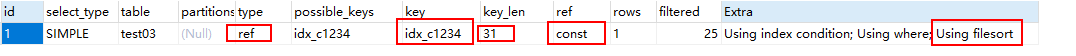Case#14：用到了两个索引

EXPLAIN SELECT * FROM test03 WHERE c1 = 'a1' AND c2 = 'a2' ORDER BY c2, c3;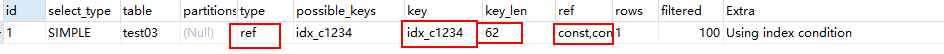Case#15：用到了两个索引

EXPLAIN SELECT * FROM test03 WHERE c1 = 'a1' AND c2 = 'a2' AND c5 = 'a5' ORDER BY c2, c3;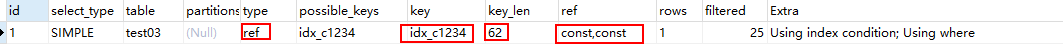Case#16：用到了两个索引

EXPLAIN SELECT * FROM test03 WHERE c1 = 'a1' AND c2 = 'a2' AND c5 = 'a5' ORDER BY c3, c2;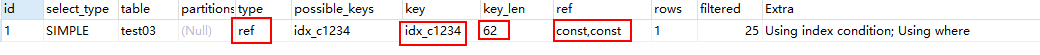Case#17：用到了一个索引（注意：此例是 GROUP BY）

EXPLAIN SELECT * FROM test03 WHERE c1 = 'a1' GROUP BY c2, c3;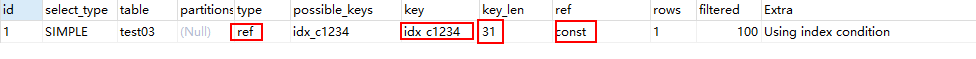Case#18：用到了一个索引（注意：GROUP BY 中的 c3 在前，c2 在后）

EXPLAIN SELECT * FROM test03 WHERE c1 = 'a1' GROUP BY c3, c2;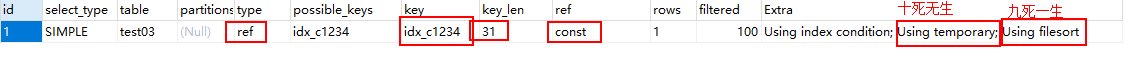• 能够用到 c1 这个索引
• GROUP BY c3, c2 等价于 ORDER BY c3, c2，而之前只有 c1 于是索引断掉了，所以出现 Using filesort（九死一生）
• GROUP BY 还需要对结果进行分组，所以产生了 Using temporary（十死无生）

Case#19：用到了两个索引

EXPLAIN SELECT * FROM test03 WHERE c1 = 'a1' AND c2 > 'a2' AND c3 = 'a3';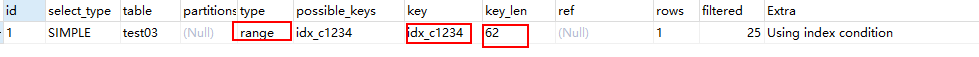Case#20：用到了三个索引（注意：中间的 c2 是 LIKE 查询，且%在右边）

EXPLAIN SELECT * FROM test03 WHERE c1 = 'a1' AND c2 LIKE 'a2%' AND c3 = 'a3';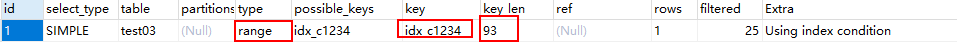Case#21：用到了一个索引（注意：中间的 c2 是 LIKE 查询，且%在左边）

EXPLAIN SELECT * FROM test03 WHERE c1 = 'a1' AND c2 LIKE '%a2' AND c3 = 'a3';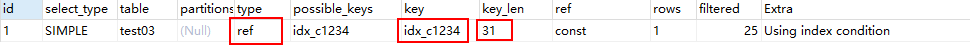Case#22：用到了一个索引（注意：中间的 c2 是 LIKE 查询，且%在两边）

EXPLAIN SELECT * FROM test03 WHERE c1 = 'a1' AND c2 LIKE '%a2%' AND c3 = 'a3';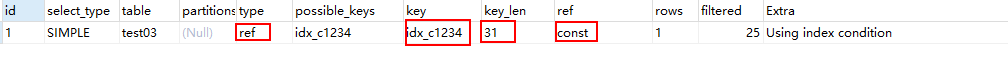Case#23：用到了三个索引

EXPLAIN SELECT * FROM test03 WHERE c1 = 'a1' AND c2 LIKE 'a2%a2%' AND c3 = 'a3';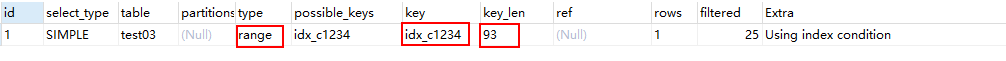posted @ 2018-04-24 15:12  cyhbyw  阅读(2582)  评论(2编辑  收藏  举报